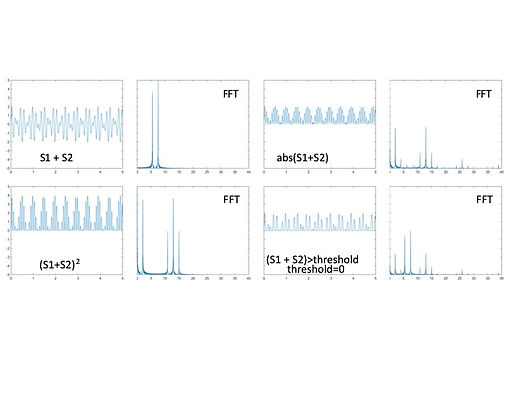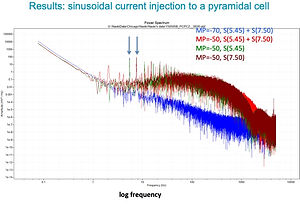top of pageWhen a non-linear system receives multiple sinusoidal inputs with different frequencies, the output has non-linear frequency components (see explanation below). For example, if two sinusoidal signals, S1 and S2, with different frequencies, f1 and f2 are given, output would have, for example, f1+f2, f1-f2, 2f1+f2 etc. In the EEG frequency tagging paradigm, this is used as a signature of neural interactions of the two signals because the emergence of these frequency components requires two conditions:

1. There are neurons that receive both inputs.

2. The signals processing by the neuron has to be non-linear.

As the input-output relationship of neurons is non-linear, if the two signals are integrated, there should be the emergent non-linear frequency components. What kind of frequencies emerge depends on the non-linear functions (figure below, right).

I investigated the properties of pyramidal neurons integrating two sinusoidal inputs and detected various emergent frequency components (figure below).bottom of page